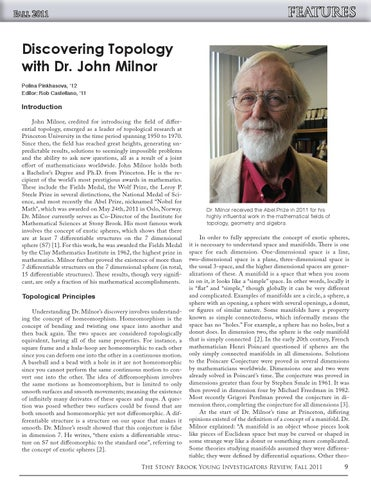features Discovering Topology with Dr. John Milnor Polina Pinkhasova, ‘12 Editor: Rob Castellano, ‘11

Introduction John Milnor, credited for introducing the field of differential topology, emerged as a leader of topological research at Princeton University in the time period spanning 1950 to 1970. Since then, the field has reached great heights, generating unpredictable results, solutions to seemingly impossible problems and the ability to ask new questions, all as a result of a joint effort of mathematicians worldwide. John Milnor holds both a Bachelor’s Degree and Ph.D. from Princeton. He is the recipient of the world’s most prestigious awards in mathematics. These include the Fields Medal, the Wolf Prize, the Leroy P. Steele Prize in several distinctions, the National Medal of Science, and most recently the Abel Prize, nicknamed “Nobel for Math”, which was awarded on May 24th, 2011 in Oslo, Norway. Dr. Milnor currently serves as Co-Director of the Institute for Mathematical Sciences at Stony Brook. His most famous work involves the concept of exotic spheres, which shows that there are at least 7 differentiable structures on the 7 dimensional sphere (S7) . For this work, he was awarded the Fields Medal by the Clay Mathematics Institute in 1962, the highest prize in mathematics. Milnor further proved the existence of more than 7 differentiable structures on the 7 dimensional sphere (in total, 15 differentiable structures). These results, though very significant, are only a fraction of his mathematical accomplishments.

Topological Principles Understanding Dr. Milnor’s discovery involves understanding the concept of homeomorphism. Homeomorphism is the concept of bending and twisting one space into another and then back again. The two spaces are considered topologically equivalent, having all of the same properties. For instance, a square frame and a hula-hoop are homeomorphic to each other since you can deform one into the other in a continuous motion. A baseball and a bead with a hole in it are not homeomorphic since you cannot perform the same continuous motion to convert one into the other. The idea of diffeomorphism involves the same motions as homeomorphism, but is limited to only smooth surfaces and smooth movements; meaning the existence of infinitely many derivates of these spaces and maps. A question was posed whether two surfaces could be found that are both smooth and homeomorphic yet not diffeomorphic. A differentiable structure is a structure on our space that makes it smooth. Dr. Milnor’s result showed that this conjecture is false in dimension 7. He writes, “there exists a differentiable structure on S7 not diffeomorphic to the standard one”, referring to the concept of exotic spheres .

Dr. Milnor received the Abel Prize in 2011 for his highly influential work in the mathematical fields of topology, geometry and algebra.

In order to fully appreciate the concept of exotic spheres, it is necessary to understand space and manifolds. There is one space for each dimension. One-dimensional space is a line, two-dimensional space is a plane, three-dimensional space is the usual 3-space, and the higher dimensional spaces are generalizations of these. A manifold is a space that when you zoom in on it, it looks like a “simple” space. In other words, locally it is “flat” and “simple,” though globally it can be very different and complicated. Examples of manifolds are a circle, a sphere, a sphere with an opening, a sphere with several openings, a donut, or figures of similar nature. Some manifolds have a property known as simple connectedness, which informally means the space has no “holes.” For example, a sphere has no holes, but a donut does. In dimension two, the sphere is the only manifold that is simply connected . In the early 20th century, French mathematician Henri Poincaré questioned if spheres are the only simply connected manifolds in all dimensions. Solutions to the Poincare Conjecture were proved in several dimensions by mathematicians worldwide. Dimensions one and two were already solved in Poincaré’s time. The conjecture was proved in dimensions greater than four by Stephen Smale in 1961. It was then proved in dimension four by Michael Freedman in 1982. Most recently Grigori Perelman proved the conjecture in dimension three, completing the conjecture for all dimensions . At the start of Dr. Milnor’s time at Princeton, differing opinions existed of the definition of a concept of a manifold. Dr. Milnor explained: “A manifold is an object whose pieces look like pieces of Euclidean space but may be curved or shaped in some strange way like a donut or something more complicated. Some theories studying manifolds assumed they were differentiable; they were defined by differential equations. Other theo-

The Stony Brook Young Investigators Review, Fall 2011

9